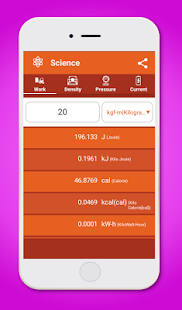Unit Converter App – This Unit Converter app was a completely free app which using in offline mode. It is a simple and easy unit converter app to handle any conversion you’ll ever need. This free Unit Converter app was mainly designed with Good UI and Simple material designed with more users friendly. In this Unit Converter app you can find almost up-to 22+ levels of categories.

This unit converter app using for all levels of oriented as like for students and profession workers. In this Simple unit converter app the Unit conversions and calculation results are displaying immediately with inputs being given. Most mainly this free simple Unit Converter app provides you almost all levels of unit values converting of around 110+ unit values, where user can make share of the results to others.
Unit Converter App – has the following features which are given on more user-friendly under different categories with the simple Unit Converter calculus.

In this free Unit Converter app on offline mode was mainly categorized as level based:

 Basic – In this basic group of our free Unit Converter app user can find the details of LENGTH ( Millimeter, Centimeter, Inch, etc.,) AREA (Square Millimeter, Square Centimeter, Acre, etc.,) WEIGHT (Microgram, Ounce, Pound etc.,) and VOLUME (Millimeter, Deciliter, Cubic Yard, etc.,)

 Living – In this living group of our free Unit Converter app user can find the details of TIME (Millisecond, Second, Year, Month, etc.,), SPEED (Meter/Second, Foot/Second, Mile/Hour, etc.,), INTEREST (It has two levels of calculation one has simple interest and other was a compound interest), TEMP (Celsius, Fahrenheit etc.,)

 Quantity – In this Quantity group of our free Unit Converter app user can find the details of FUEL (Meter/Liter, Kilometer/Liter, etc.,), BINARY (Binary, Octal, Decimal, etc.,), BLOOD (Milligrams/DeciLitre, Mill moles/Liter, etc.,), DATA (Bit, Byte, Kilobyte, etc.,).

 Science – In this Quantity group of our free Unit Converter app user can find the details of WORK (Joule, Kilo Joule, Calorie, etc.,), DENSITY (Milligram/Cubic Millimeter, Milligram/ Cubic Centimeter, etc.,), PRESSURE ( Atmosphere, Pascal, etc.,), CURRENT (Pico Ampere, Nano Ampere, Micro Ampere, etc.,)

It’s a very simple and easy Unit Converter App with more user-friendly and well material designed, Make use of this free offline Unit Converter App.

Screenshots

•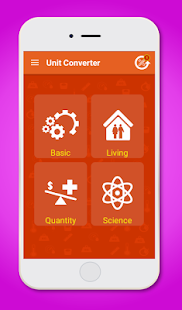•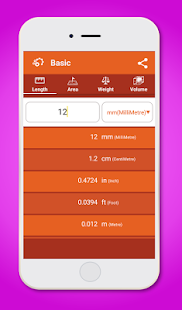•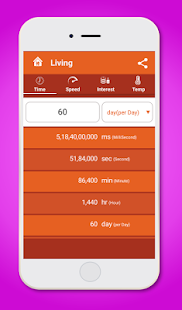•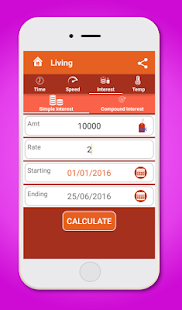•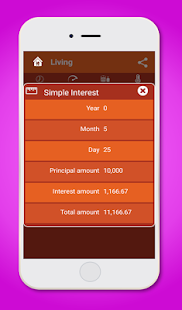•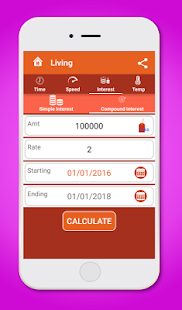•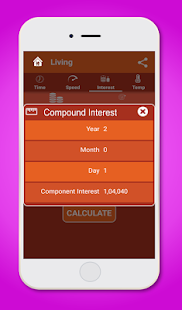•